# C/C++面试知识点总结（一）

1.C/C++

3.数据结构与算法

4.计算机网络

5.操作系统

6.数据库

1.纯属个人YY

1.C/C++

(1).struct大小的确定

struct test
{
int a;
char b;
int c;
}TEST;


struct test
{
int a;
char b;
char d;
char e;
char f;
int c;
}TEST;


struct test
{
int a;
char b;
int c;
char d;
}TEST;


struct test
{
char a;
}TEST;


struct test
{
char a;
char b;
}TEST;


(2).strlen、strcpy的实现

int _strlen(const char* str)
{
if (!str || *str == 0) return 0;
int len = 0;
while (*str++)++len;
return len;
}

char* _strcpy(char* d, const char* s)
{
if (!s || *s == 0) return d ? &(*d = 0) : NULL; //防止d未初始化乱码
char* td = d;
if (d) while (*d++ = *s++); //防止d为NULL
return td;
}



(3).memcpy的实现以及如何防止内存重叠造成的错误。

void* Memcpy(void* des, const void* src, size_t count)
{
if (!src || count <= 0) return des;
char* td = (char*)des;
const char* ts = (const char*) src;
while (count--) *td++ = *ts++;
return des;
}


	char* p = new char;
memcpy(p, "12345", 10);
printf("%s\n", p);
Memcpy(p + 1, p, 4);
printf("%s\n", p);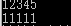void* Memmove(void* des,const void* src,size_t count)
{
if (!src || count <= 0) return des;
char* td = (char*)des;
const char* ts = (char*)src;
//如果源地址 + count 小于目的地址，说明，内存无重叠，进行正向拷贝
if (ts + count < td)
{
while (count--) *td++ = *ts++;
}
//否则有内存重叠，进行逆向拷贝
else
{
char* ttd = td + count - 1;
const char* tts = ts + count - 1;
while (count--) *ttd-- = *tts--;
}
return des;
}


	char* p = new char;
memcpy(p, "12345", 10);
printf("%s\n", p);
Memmove(p + 1, p, 4);
printf("%s\n", p);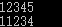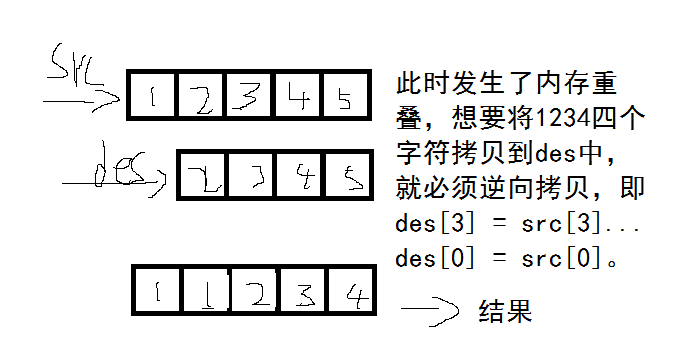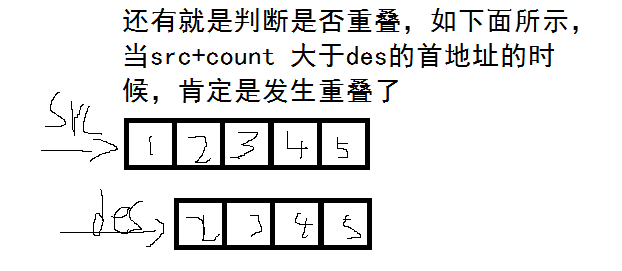(4).全局变量、局部变量、静态变量的作用域以及存放的内存区域。

(5).static关键字的作用

a.在函数体内部定义变量，该变量从程序开始到结束只会分配一次内存，当再次进入该函数的时候，其值不变，仍为上次退出时的值

int f()
{
static int i = 0;
return ++i;
}

int main()
{
cout << f() << f() << f() << endl;
return 0;
}


b.模块内的static定义的变量和函数不能被外部引用，唯一的办法是通过一个间接变量或者函数来引用才行。

A.cpp
static char C = 'A';
char c = C;
static int F()
{
return 5;
}
int ff()
{
return F();
}

B.cpp
int main()
{
extern int ff();
extern char c;
cout << ff() << endl;
cout << c << endl;
return 0;
}


c.类中定义的static变量属于整个类，即类成员变量，与对象无关,只会在运行的时候创建一次。

d.类中定义的static函数属于整个类，及类成员函数，与对象无关，所以不能在静态函数里面使用this指针，这个是对象独有的。

例子：
class TEST
{
public:
static int m_i;
static int f() { return m_i; } //静态成员函数只能引用静态成员变量
};
int TEST::m_i = 6;
int main()
{
cout << TEST::f() << endl;
return 0;
}


(6).const关键字的作用
a.定义普通变量的时候，只能初始化一次，以后不可再修改其值。
b.定义指针变量时，再类型前，则值不能改，再类型后，则其地址不能改，若两个都有，则两者都不能改。

int a = 2, b = 3;
const int* p1 = &a;
*p1 = b; //值不可以修改
p1 = &b; //地址可以修改
int* const p2 = &a;
*p2 = b; //值可以修改
p2 = &b; //地址不可以修改


c.在函数形参声明中，使用const，可以防止传进来的参数被修改。
d.在类中使用const定义的函数，在函数内部不能修改成员变量的值,但是可以修改传进来的形参值，但是一般不这么用。

(7).sizeof是运算符而不是函数，计算变量或者结构体大小的时候可以不加括号，但是计算类型一定要加，所以计算什么都加就对了。

(8).数组地址问题

int a[] = { 1,2,3,4,5 };
cout << "a: " << &a << endl;
cout << "a: " << endl;
cout << "(a + 1): " <<&a + 1 << endl;
cout << "(a + 1): " << a + 1 << endl;
cout << "&a + 1: " << &a + 1 << endl;


(9).如何将浮点数分解为整数和小数部分

a.强转为int即为整数部分，然后再减去整数部分即可。

double Modf(double x, int* y)
{
*y = (int)x;
return x - *y;
}


b.直接使用库函数，double modf(double x,double* y);

(10).其它一样，只改变一样，不能使函数重载的是

(11).C的结构体和C++的结构体的区别

a.C结构体内部不允许有函数存在，C++允许
b.内部成员变量权限不同，C的只能是public，C++的可以有三种。
c.C结构体不可以继承，C++可以继承结构体或者类

(12).浅拷贝和深拷贝的原理

(13).不能重载的5个运算符

.(成员访问运算符)
->(成员指针运算符)
::(作用域解析运算符)
?(条件运算符)
sizeof运算符

(14)常见的不能声明为虚函数的有哪些？

(15)C++的静态多态和动态多态

(16)C++虚函数的原理

class A
{
public:
virtual void f1() { cout << "A...f1()" << endl; }
};

class B :public A
{
public:
void f1() { cout << "B...f1()" << endl; }
};

int main()
{

A* a = new A;   //因为不是抽象类，所以可以实例化
B* b = new B;   //直接用子类自己实例化
A* pB = new B;  //用基类指针指向子类

return 0;
}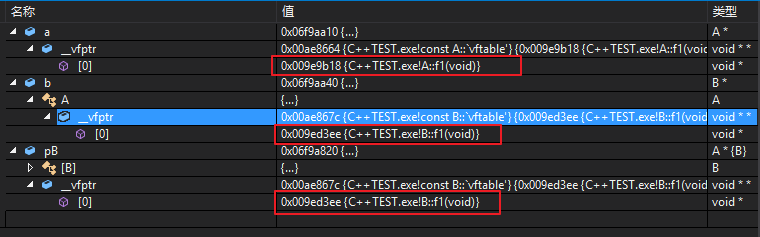(17)C++虚函数占用类的大小

(18)new与malloc的区别

(19)C++中有哪几种数据存储区？

(20)什么是栈溢出？哪些情况下比较容易出现栈溢出？

(21)“#include”后面跟引号与尖括号的区别？

(22)gcc和g++的区别

(23)类成员函数的重载、覆盖和重写区别

(24)构造函数为什么不能是虚函数

(25)printf("%d,%d\n",i++,i++),若i=0,则结果输出什么。

_acme_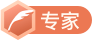CSDN认证博客专家 JAVA

12-01
09-194万+08-143541
07-194万+
09-24527
04-111万+
07-011万+
01-30
08-21185
06-041763
04-013万+
©️2020 CSDN 皮肤主题: Age of Ai 设计师:meimeiellie